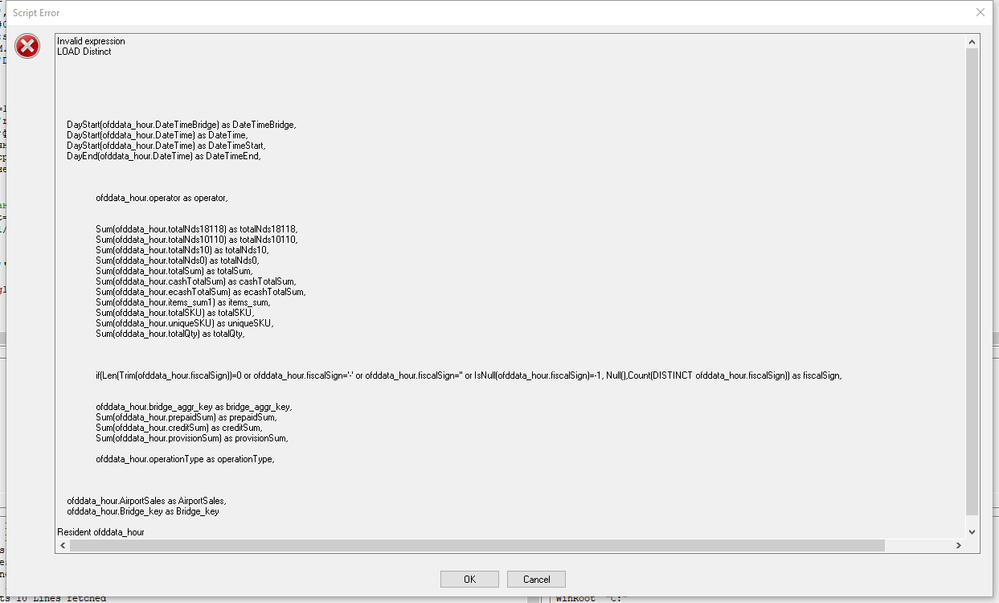# New to QlikView

Discussion board where members can get started with QlikView.

Announcements
Leverage your QlikView investment to modernize BI – see how! Join Group
cancel
Showing results for
Did you mean:
HighlightedCreator

## Null value Invalid expression

Hi, All

I have a script expression

if(Len(Trim(ofddata_hour.fiscalSign))=0 or ofddata_hour.fiscalSign='-' or ofddata_hour.fiscalSign='' or IsNull(ofddata_hour.fiscalSign), Null(),Count(DISTINCT ofddata_hour.fiscalSign)) as fiscalSign

which don't work.

Script returns an error "Invalid expression"

Why? Any idea?

Many thanks,

Labels (4)

• ### Null()

1 Solution

Accepted Solutions
HighlightedSpecialist III

## Re: Null value Invalid expression

try below expression

count(DISTINCT  if(Len(Trim(ofddata_hour.fiscalSign))=0 or ofddata_hour.fiscalSign='-' or ofddata_hour.fiscalSign='' or IsNull(ofddata_hour.fiscalSign)=0, Null() , ofddata_hour.fiscalSign)) as fiscalSign,

6 Replies
HighlightedMVP

## Re: Null value Invalid expression

What if you add a comparison for the IsNull?

if(Len(Trim(ofddata_hour.fiscalSign))=0 or ofddata_hour.fiscalSign='-' or ofddata_hour.fiscalSign='' or IsNull(ofddata_hour.fiscalSign)=-1, Null(),Count(DISTINCT ofddata_hour.fiscalSign)) as fiscalSign

Note: IsNull evaluates to -1 for true and 0 for false.

HighlightedCreator

## Re: Null value Invalid expression

unfortunately did not help...HighlightedSpecialist III

## Re: Null value Invalid expression

HI,

issue might be because  of Count function usage in the Script with out any aggregation.

try the same expression in any chart

thanks,

sasi

HighlightedCreator

## Re: Null value Invalid expression

My script code below.

I used Group by .  With expression Count(DISTINCT ofddata_hour.fiscalSign) as fiscalSign

if(Len(Trim(ofddata_hour.fiscalSign))=0 or ofddata_hour.fiscalSign='-' or ofddata_hour.fiscalSign='' or IsNull(ofddata_hour.fiscalSign)=0, Null(),Count(DISTINCT ofddata_hour.fiscalSign)) as fiscalSign

ofddata_day:
Qualify *;
DayStart(ofddata_hour.DateTimeBridge) as DateTimeBridge,
DayStart(ofddata_hour.DateTime) as DateTime,
DayStart(ofddata_hour.DateTime) as DateTimeStart,
DayEnd(ofddata_hour.DateTime) as DateTimeEnd,
ofddata_hour.operator as operator,
Sum(ofddata_hour.totalNds18118) as totalNds18118,
Sum(ofddata_hour.totalNds10110) as totalNds10110,
Sum(ofddata_hour.totalNds10) as totalNds10,
Sum(ofddata_hour.totalNds0) as totalNds0,
Sum(ofddata_hour.totalSum) as totalSum,
Sum(ofddata_hour.cashTotalSum) as cashTotalSum,
Sum(ofddata_hour.ecashTotalSum) as ecashTotalSum,
Sum(ofddata_hour.items_sum1) as items_sum,
Sum(ofddata_hour.totalSKU) as totalSKU,
Sum(ofddata_hour.uniqueSKU) as uniqueSKU,
Sum(ofddata_hour.totalQty) as totalQty,
// if(Len(Trim(ofddata_hour.fiscalSign))=0 or ofddata_hour.fiscalSign='-' or ofddata_hour.fiscalSign='' or IsNull(ofddata_hour.fiscalSign)=0, Null(),Count(DISTINCT ofddata_hour.fiscalSign)) as fiscalSign,
Count(DISTINCT ofddata_hour.fiscalSign) as fiscalSign,
ofddata_hour.bridge_aggr_key as bridge_aggr_key,
Sum(ofddata_hour.prepaidSum) as prepaidSum,
Sum(ofddata_hour.creditSum) as creditSum,
Sum(ofddata_hour.provisionSum) as provisionSum,
ofddata_hour.operationType as operationType,
ofddata_hour.AirportSales as AirportSales,
ofddata_hour.Bridge_key as Bridge_key
Resident ofddata_hour
Group by DayStart(ofddata_hour.DateTimeBridge), DayStart(ofddata_hour.DateTime), DayEnd(ofddata_hour.DateTime),ofddata_hour.operator, ofddata_hour.bridge_aggr_key, ofddata_hour.operationType, ofddata_hour.AirportSales, ofddata_hour.Bridge_key;
Unqualify *;

HighlightedSpecialist III

## Re: Null value Invalid expression

try below expression

count(DISTINCT  if(Len(Trim(ofddata_hour.fiscalSign))=0 or ofddata_hour.fiscalSign='-' or ofddata_hour.fiscalSign='' or IsNull(ofddata_hour.fiscalSign)=0, Null() , ofddata_hour.fiscalSign)) as fiscalSign,

HighlightedCreator

## Re: Null value Invalid expression

look like what i need...

Thanks!